# Chapter 12 Surface Areas and Volumes NCERT Exemplar Solutions Exercise 12.4 Class 10 MathsChapter Name NCERT Maths Exemplar Solutions for Chapter 12 Surface Areas and Volumes Exercise 12.4 Book Name NCERT Exemplar for Class 10 Maths Other Exercises Exercise 12.1Exercise 12.2Exercise 12.3 Related Study NCERT Solutions for Class 10 Maths

### Exercise 12.4 Solutions

1. A solid metallic hemisphere of radius 8 cm is melted and recasted into a right circular cone of base radius 6 cm. Determine the height of the cone.

Solution

For hemisphere,
We know that,
Volume of hemisphere = (2/3)πr3 , where, r = radius of hemisphere
Volume of given hemisphere = (2/3) × π × 83
= (1024/3)π cm3
Now,
For the cone that is recast from a hemisphere,
Base radius, r = 6 cm
We also know that,
Volume of cone = (1/3)πr2 h, where, r is base radius and h is the height of cone.
Volume of cone = (1/3)π(6)2 h
= 12πh
According to the question, we know that,
The volume remains same, when a body is reformed to another body
Volume of cylinder = Volume of cone
12πh = 1024π/3
h = 28.44 cm

2. A rectangular water tank of base 11 m × 6 m contains water upto a height of 5 m. If the water in the tank is transferred to a cylindrical tank of radius 3.5 m, find the height of the water level in the tank.

Solution

Volume of water in tank = volume of cuboidal tank up to a height of 5 m
According to the question,
For cuboidal tank
Length, l = 11 m
Height, h = 5m
We know that the equation to find the volume of the tank,
Volume of tank = lbh, where, l, b and h are the length, breadth and height of tank respectively
Volume of water = 11(6)(5) = 330 m3
We also know that,
Base radius of cylindrical tank, r = 3.5 m
Let the height till which the cylindrical tank is filled = h m
Hence, using the formula,
Volume of a cylinder = πr2 h,
where r is base radius and h is the height of cylinder
Volume of water in cylindrical tank = π(3.5)2 h
330 m3 = 22/7 × 3.5 ×3.5 × h
⇒ h = 8.57 m
Hence, the height till which the cylindrical tank is filled = 8.57 m

3. How many cubic centimetres of iron is required to construct an open box whose external dimensions are 36 cm, 25 cm and 16.5 cm provided the thickness of the iron is 1.5 cm. If one cubic cm of iron weighs 7.5 g, find the weight of the box.

Solution

Let the length (l), breath (b), and height (h) be the external dimension of an open box and thickness be x.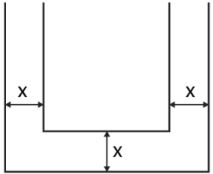The volume of metal used in box = Volume of external box – Volume of internal box
Consider external box,
Length, l = 36 cm
Height, h = 16.5 cm
We know that the equation of the volume of cuboid is given by,
Volume of cuboid = lbh, where, l, b and h are the length, breadth and height of tankbrespectively
Volume of external box = 36(25)(16.5)
= 14850 cm3
Since the box is open from top,
Consider internal box,
The thickness of two sides is reduced as follows,
Length, l‟ = Length of external box – 2(thickness of box)
= 36 – 2(1.5)
= 33 cm
= 25 – 2(1.5)
= 22 cm
Height,
h‟ = Height of external box – thickness of box
= 16.5 – 1.5
= 15 cm
Volume of internal box = 33(22)(15)
= 10890
Volume of metal in box = 14850 – 10890 = 3960 cm3
1 cm3 weighs 7.5 g.
3960 cm3  weighs 3960(7.5) = 29,700 g
Therefore, the weight of box is 29,700 g i.e. 29.7 kg

4. The barrel of a fountain pen, cylindrical in shape, is 7 cm long and 5 mm in diameter. A full barrel of ink in the pen is used up on writing 3300 words on an average. How many words can be written in a bottle of ink containing one fifth of a litre?

Solution

Let us first calculate the volume of barrel of pen that is of cylindrical shape
Consider barrel,
Since 1cm = 10 mm
Base diameter = 5 mm
= 0.5 cm
Base radius, r = 0.25 cm
Height, h = 7 cm
We know that,
Volume of a cylinder = πr2 h
Volume of barrel = π (0.25)2 7
Volume of barrel = 22/7 × 0.25 × 0.25 × 7
= 1.375 cm3
Hence, according to the question,
1.375 cm3  of ink can write 3300 words
No of words that can be written by 1 cm3 of ink = 3300/1.375
= 2400 words
1/5th of a liter = 0.2 L
We know that,
1 L = 1000 cm3
⇒ 0.2 L = 200 cm3
So, no of words that can be written by 200 cm3  = 2400(200)
= 480000 words
Therefore, 1/5th of a liter ink can write 480000 words.

5. Water flows at the rate of 10m/minute through a cylindrical pipe 5 mm in diameter. How long would it take to fill a conical vessel whose diameter at the base is 40 cm and depth 24 cm?
Solution
Let the time taken by pipe to fill vessel = t minutes
Since water flows 10 m in 1 minute, it will flow 10t meters in t minutes.
According to the question,
Volume of conical vessel = Volume of water that passes through pipe in t minutes
Consider conical pipe,
Base Diameter = 40 cm
Base radius, r = 20 cm
Height, h = 24 cm
We know that the volume of cone = (1/3)πr2 h
Volume of conical vessel = (1/3)π(20)2 (24)
= 3200π cm3
Consider cylindrical pipe
Base diameter = 5 mm
= 0.5 cm
r = 0.25 cm
Water covers 10t m distance in pipe,
Hence, we get ,
Height .
h = 10 t m = 1000t cm
We also know that,
Volume of a cylinder = πr2 h
Volume of water passed in pipe  = π(0.25)2 (1000t)
= 62.5t cm3
So, we have
62.5tπ = 3200
⇒ 62.5t = 3200
⇒ t = 51.2 minutes
We know that,
0.2 minutes  = 0.2(60) seconds
= 12 seconds
Therefore,
t = 51 minutes 12 seconds

6. A heap of rice is in the form of a cone of diameter 9 m and height 3.5 m. Find the volume of the rice. How much canvas cloth is required to just cover the heap?
Solution
According to the question,
Consider conical heap,
Base Diameter = 9 cm
So,
Base radius, r = 4.5 cm
Height, h = 3.5 cm
We know that,
Slant height,
l = √(h2 + r2 )
= √[(4.5)2 + (3.5)2 ]
= 5.7 cm
The equation of volume of cone = (1/3)πr2 h
We know that,
Volume of rice = Volume of conical heap
Volume of rice = (1/3)π(4.5)2 (3.5)
= 74.25 cm3
We also know that,
Canvas requires to just cover heap = Curved surface area of conical heap
And curved surface area of a cone = πrl
Therefore, the canvas required = π(4.5)(5.7) = 80.61 cm2 [appx.]

7. A factory manufactures 120000 pencils daily. The pencils are cylindrical in shape each of length 25 cm and circumference of base as 1.5 cm. Determine the cost of colouring the curved surfaces of the pencils manufactured in one day at Rs 0.05 per dm2 .
Solution
The shape of pencil = cylinder.
Let the radius of base = r cm
Circumference of base = 1.5 cm
Circumference of circle is 2πr = 1.5 cm
r = (1.5/2)π  cm
According to the question,
Height, h = 25 cm
We know that,
Curved surface area of cylinder = 2πrh
Curved surface area of pencil = 2π(1.5π/)25
= 37.5 cm2
1 cm = 0.1 dm
1 cm2 = 0.01 dm2
⇒ 37.5 cm2 = 0.375 dm2
Cost for colouring 1 dm2 = Rs. 0.05
Cost for colouring 0.375 dm2 (i.e. 1 pencil) = Rs. 0.01875
Cost for colouring 120000 pencils = 120000 × 0.01875
= Rs. 2250

8. Water is flowing at the rate of 15 km/h through a pipe of diameter 14 cm into a cuboidal pond which is 50 m long and 44 m wide. In what time will the level of water in pond rise by 21 cm?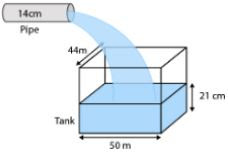Solution
Let the time taken by pipe to fill pond = t hours
Water flows 15 km in 1 hour, so it will flow 15t meters in t hours.
We know that,
Volume of cuboidal pond up to height 21 cm = Volume of water that passes through pipe in "t" hours
Considering cuboidal pond,
Length, l = 50 m
height, h = 21 cm
= 0.21 m
We know that,
Volume of tank  = lbh
Volume of water = 50(44)(0.21)
= 462 m3
Considering cylindrical pipe
Base diameter = 14 cm
Base radius, r = 7 cm
= 0.07 m
Height, h = 15t km
= 15000t m
We also know that,
Volume of a cylinder  = πr2 h
Volume of water passed in pipe = π(0.07)2 (15000t)
= (22/7) × 0.07 × 0.07 × 15000t
= 231t cm3
So, we have
231t = 462
⇒ t = 2 hours
Time required to fill tank up to a height of 25 cm is 2 hours.

9. A solid iron cuboidal block of dimensions 4.4 m × 2.6 m × 1m is recast into a hollow cylindrical pipe of internal radius 30 cm and thickness 5 cm. Find the length of the pipe.
Solution
Considering cuboidal block
Length l = 4m
Height, h = 1 m
We know that,
Volume of tank  = lbh
Volume of cuboid = 4.4 × 2.6 × 1
= 11.44 m3
We know that,
The volume remains same when a body is recast to another body.
According to the question,
Volume of cylindrical pipe  = 11.44 m3
Considering pipe or the hollow cylinder
r2 = 30 cm
= 0.3 m
Thickness = 5 cm
= 30 + 5
= 35 cm
= 0.35m
Let the length of pipe = h
We know that,
Volume of a hollow cylinder = πh(r12 - r22)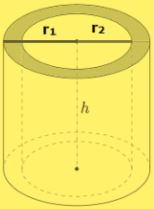Hence,
Volume of pipe  = πh[(0.35)2 - (0.3)2]
So, the length of pipe is 112m.

10. 500 persons are taking a dip into a cuboidal pond which is 80 m long and 50 m broad. What is the rise of water level in the pond, if the average displacement of the water by a person is 0.04m3  ?
Solution
According to the question,
Average displacement by a person = 0.04 m3
Average displacement by 500 persons = 500 × 0.04
= 20 m3
Hence, the volume of water raised in pond = 20 m3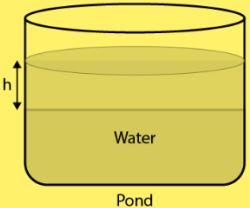It is also given that,
Length of pond, l  80m
Breadth of pond, b = 50 m
Height = 4
Volume of water raised in pond = 80 × 50 × h
20m3 = 4000h
⇒ h = 0.005 m = 0.5cm
Therefore, raise in the height of water = 0.5 cm

11. 16 glass spheres each of radius 2 cm are packed into a cuboidal box of internal dimensions 16 cm × 8 cm × 8 cm and then the box is filled with water. Find the volume of water filled in the box.
Solution
Given,
Dimensions of the cuboidal = 16 cm × 8 cm × 8 cm
Volume of the cuboidal = 16 × 8 × 8 = 1024 cm3
Also, given radius of one glass sphere = 2 cm
Volume of one glass sphere,
= (4/3)πr3
= (4/3)π(2)3
= 33.523 cm3
Volume of 16 glass sphere = 16 × 33.523
= 536.37 cm3
Required volume of water = Volume of the cuboidal - Volume of 16 glass sphere
= 1024 - 536.37
= 487.6 cm3

12. A milk container of height 16 cm is made of metal sheet in the form of a frustum of a cone with radii of its lower and upper ends as 8 cm and 20 cm respectively. Find the cost of milk at the rate of Rs. 22 per litre which the container can hold.
Solution
Given,
Height of milk container (h) = 16 cm,
Radius of lower end of milk container (r) = 8 cm
Radius of upper end of milk container (R) = 20 cm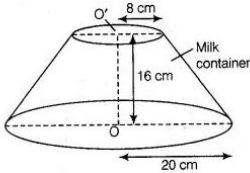Volume of milk container made of metal sheet in form of frustum of a cone,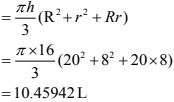Cost of milk per litre = Rs.22
For,
10.45942 L = 22 × 10.45942
= Rs. 230.12
Hence, the required cost of milk is ₹ 230.12.

13. A cylindrical bucket of height 32 cm and base radius 18 cm is filled with sand. This bucket is emptied on the ground and a conical heap of sand is formed. If the height of the conical heap is 24 cm, find the radius and slant height of the heap.
Solution
Given,
Radius of the base of the bucket = 18 cm
Height of the bucket = 32 cm
So,
Volume of the sand in cylindrical bucket = πr2 h
= π(18)2 × 32
= 10368 π
Also,
Height of the conical heap(h) = 24 cm
Let radius of heap be r cm.
So,
Volume of sand in heap (cone) = 8πr2
According to the question,
Volume of the sand in cylindrical bucket = Volume of the sand in conical heap
10368 π = 8πr2
On solving,
r = 36 cm
Let, the slant height of heap be l
l2 = h2 + r2
= 242 + 362
= 43.267 cm
Hence, radius of conical heap of sand = 36 cm
Slant height of conical heap  = 43.267 cm

14. A rocket is in the form of a right circular cylinder closed at the lower end and surmounted by a cone with the same radius as that of the cylinder. The diameter and height of the cylinder are 6 cm and 12 cm, respectively. If the slant height of the conical portion is 5 cm, find the total surface area and volume of the rocket
[Use π = 3.14].
Solution
Since, rocket is the combination of a right circular cylinder and a cone.
Given, diameter of the cylinder = 6 cm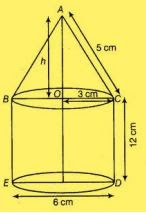Radius of cylinder = 3 cm
Height = 12 cm
Volume of cylinder ,
= πr2 h
= 3.14 × 32 × 12
= 339.12 cm3
Curved surface area of cylinder = 2× 3.14 × 3 × 12 = 226.08
Now,
Height of cone  = 4 cm
Radius of cone = 3 cm
So,
Volume of cone = (1/3)πr2 h
= (1/3)π × 32 × 4
= 37.68 cm3
Also,
C.S.A. = πrl
= π ×3 × 5
= 47.1
Therefore,
Total volume of rocket = 339.12 + 37.68
= 376.8 cm3
Now,
Total surface area of rocket = CSA of cone + CSA of cylinder + Area of base of cylinder
= 47.1 + 226.08 + 28.26
= 301.44 cm2

15. A building is in the form a cylinder surmounted by a hemispherical vaulted dome and contains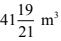of air. If the internal diameter of dome is equal to its total height above the floor, find the height of the building?
Solution
Let total height of the building = Internal diameter of the dome = 2r m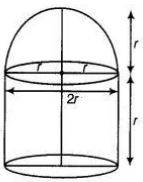Height of cylinder = 2r - r  = r m
So,
Volume of cylinder = πr2 × r
Also,
Volume of hemispherical dome = (2/3)πr3
Therefore,
Total volume of building  = Volume of cylinder + Volume of hemispherical dome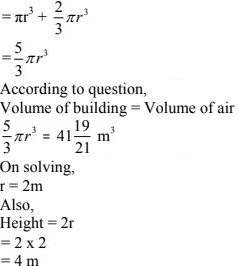16. A hemispherical bowl of internal radius 9 cm is full of liquid. The liquid is to be filled into cylindrical shaped bottles each of radius 1.5 cm and height 4 cm. How many bottles are needed to empty the bowl?
Solution
Given,
Radius of hemispherical bowl, r = 9 cm
R = 1.5 cm and
h = 4 cm
Number of required cylindrical bottles = (Volume of hemispherical bowl)/(Volume of one cylindrical bottle)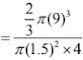= 54

17. A solid right circular cone of height 120 cm and radius 60 cm is placed in a right circular cylinder full of water of height 180 cm such that it touches the bottom. Find the volume of water left in the cylinder, if the radius of the cylinder is equal to the radius of the cone.
Solution
(i)Whenever we placed a solid right circular cone in a right circular cylinder with full of water, then volume of a solid right circular cone is equal to the volume of water failed from the cylinder.
(ii) Total volume of water in a cylinder is equal to the volume of the cylinder.
(iii) Volume of water left in the cylinder = Volume of the right circular cylinder – volume of a right circular cone.
Now, given that
Height of a right circular cone = 120 cm
Radius of a right circular cone = 60 cm
Volume of right circular cone,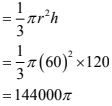Volume of right circular cone = Volume of water filled from the cylinder
= 144000π
Now,
Height of right circular cylinder = 180 cm
Volume of right circular cylinder = πr2 h
= π(60)2 × 180
= 648000π
Hence, the required volume of water left in the cylinder is 1.584 m3.

18. Water flows through a cylindrical pipe, whose inner radius is 1 cm, at the rate of 80 cm/sec in an empty cylindrical tank, the radius of whose base is 40 cm. What is the rise of water level in tank in half an hour?
Solution
Given,
Radius of tank, r1 = 40 cm
Let height of water level in tank in half an hour = 1 cm.
Also,
Internal radius of cylindrical pipe, r2 = 1 cm
Speed of water = 80 cm/s (in 1 water flow = 80 cm)
In 30 (min) water flow = 80 × 60 × 30 = 144000 cm
According to the question,
Volume of water in cylindrical tank = Volume of water flow from circular pipe
πr12 h1 = πr22 h2
⇒ 402 × h1 = 12 × 144000
⇒ h1 = 90 cm
Hence, the level of water in cylindrical tank rises 90cm in half an hour.

19. The rain water from a roof of dimensions 22 m 20 m drains into a cylindrical vessel having diameter of base 2 m and height 3.5 m. If the rain water collected from the roof just fill the cylindrical vessel, then find the rainfall in cm.
Solution
Given,
Length of roof = 22m and
Breadth of roof = 20 m
Let the rainfall be a cm.
Volume of water on roof = 22 × 20 × a/100
= 22a/5
Now,
Volume of cylindrical vessel  = 22/7 × 1 × 1 × 7/2
= 11 m3
According to question,
Volume of water on roof = Volume of cylindrical vessel
22a/5 = 11
⇒ a = 2.5 cm
Hence, the rainfall is 2.5 cm

20. A pen stand made of wood is in the shape of a cuboid with four conical depressions and a cubical depression to hold the pens and pins, respectively. The dimension of the cuboid are 10 cm, 5 cm and 4 cm. The radius of each of the conical expressions is 0.5 cm and the depth is 2.1 cm. The edge of the cubical depression is 3 cm. Find the volume of the wood in the entire stand.
Solution
Given that, length of cuboid pen stand (l) = 10 cm
Breadth of cubiod pen stand (b) = 5 cm
Height of cuboid pen stand (h) = 4 cm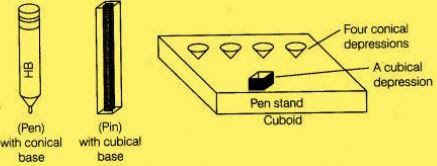Volume of cuboidal pen stand  = 10 × 5 × 4
= 200 cm3
Radius of conical depression = 0.5 cm
Depth = 2.1 cm
Volume of conical depression,
= (1/3)πr2 h
= (1/3) × (22/7) × 0.5 × 0.5 × 2.1
= 0.55 cm3
So,
Volume of four conical depression = 4 × 0.55
= 11/5  cm3
Now,
Edge of cube = 3 cm
Volume of cube = 27 cm3
Therefore,
Volume of wood in entire pen stand = Volume of cuboidal pen stand - Volume of four conical depression - Volume of cube
= 200 - (11/5) - 27
= 170.8 cm3
So, the required volume of the wood in the entire stand is 170.8 cm3.